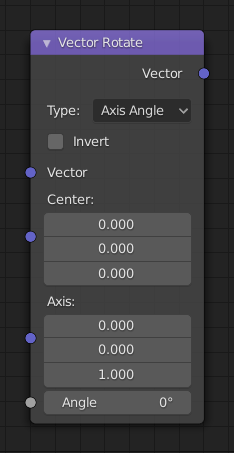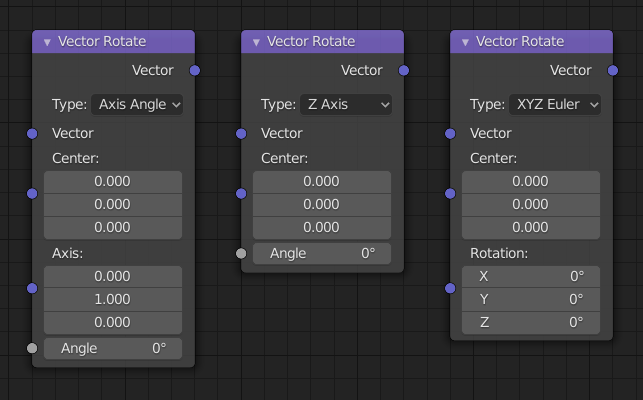# 矢量旋转节点¶Vector Rotate Node.

The Vector Rotate Node provides the ability to rotate a vector around a pivot point (Center).

## 输入¶

Vector to be rotated.

Point to rotate around.

## 属性¶

Rotates the vector around an axis defined by the Axis input vector and the amount of rotation is defined by the Angle input.

X, Y, Z Axis

Rotates the vector around the defined axis and the amount of rotation is defined by the Angle input.

Rotates the vector about the Center and defined by the Rotation input vector to control the amount of rotation on each axis.

Inverts the rotation angle.

## 输出¶

The rotated vector.

## 示例¶Vector Rotate node example.